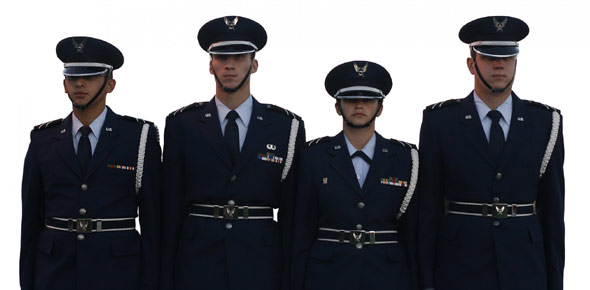# 3D1X3 Vol 3 CDC Ed2

100 Questions | Total Attempts: 127Settings3d1x3 Vol 3 CDC Ed2

• 1.
(401) In the tropospheric scatter mode, the high power amplifier amplifies the radio frequency to
• A.

1 milliwatt.

• B.

1 watt.

• C.

100 watt.

• D.

1 kilowatt.

• 2.
(401) Which type of diversity requires two antennas, two receivers, and two transmitters?
• A.

Polarization.

• B.

Crossband.

• C.

Frequency.

• D.

Space.

• 3.
(401) The MD–1026 digital data modem can interface up to four groups of, which type of signals?
• A.

Balanced non-return-to-zero (NRZ).

• B.

Conditioned diphase.

• C.

Unbalanced NRZ.

• D.

Isochronous.

• 4.
(401) The trunk group multiplexer TD–1236 can multiplex up to
• A.

One group of the 512 Kbps data rate family.

• B.

One group of the 576 Kbps data rate family.

• C.

Four groups of the 512 Kbps data rate families or 576 Kbps data rate families.

• D.

Four groups of the 12 Kbps data rate families and 576 Kbps data rate families.

• 5.
(401) Digital voice orderwire traffic that originates at the AN/TRC–170’s voice orderwire control unit, is encrypted by the
• A.

KY–68.

• B.

KY–58.

• C.

KG–84.

• D.

KG–81.

• 6.
(401) Which jack field in the AN/TRC–170’s baseband patch panel provides timing and data connections?
• A.

Middle.

• B.

Center.

• C.

Upper.

• D.

Lower.

• 7.
(401) The tropospheric scatter modem processes mission traffic at data rates of 128 through 4,096 Kbps, within which data rate family?
• A.

512 Kbps.

• B.

576 Kbps.

• C.

1,024 Kbps.

• D.

1,152 Kbps.

• 8.
(401) Which unit in the AN/TRC–170 terminal contains the rubidium standard?
• A.

Electrical synthesizer.

• B.

Frequency subsystem.

• C.

Tropospheric scatter modem.

• D.

Transmitter-amplifier converter.

• 9.
(401) The quick reaction antenna for the V3 AN/TRC–170 terminal uses how many linearly dualpolarized antennas?
• A.

Two 6-foot-diameter.

• B.

Four 6-foot-diameter.

• C.

Two 9.5-foot-diameter.

• D.

Four 9.5-foot-diameter.

• 10.
(402) The Tropo/Satellite Support Radio frequency coverage extends from
• A.

4.4 to 5.25 GHz.

• B.

7.25 to 8.4 GHz.

• C.

14.4 to 15.25 GHz.

• D.

17.25 to 18.4 GHz.

• 11.
(402) Which maximum distance in miles can a Tropo/Satellite Support Radio operate with the 2- foot antenna?
• A.

9

• B.

24

• C.

90

• D.

240

• 12.
(402) What is the maximum distance in feet the Tropo/Satellite Support Radio’s radio frequency and baseband assemblies can be separated?
• A.

50

• B.

100

• C.

150

• D.

200

• 13.
(402) In the Tropo/Satellite Support Radio’s baseband assembly, a 1-volt peak-to-peak input signal results in how much frequency deviation in the transmitter?
• A.

±4 MHz.

• B.

Less than ±4 MHz.

• C.

More than ±4 MHz.

• D.

No signal deviation.

• 14.
(402) Which frequency is the intermediate frequency of the Tropo/Satellite Support Radio?
• A.

70 MHz with a 10 MHz bandpass.

• B.

70 MHz with a 20 MHz bandpass.

• C.

700 MHz with a 10 MHz bandpass.

• D.

700 MHz with a 20 MHz bandpass.

• 15.
(403) What is the inclination angle of a polar orbit?
• A.

0°.

• B.

45°.

• C.

90°.

• D.

180°.

• 16.
(403) What is the procedure called when keeping a satellite in a desired position, in orbit and within acceptable limits?
• A.

Shift.

• B.

Period.

• C.

Air drag.

• D.

Station keeping.

• 17.
(404) Which satellite reflects signals transmitted toward it?
• A.

Active.

• B.

Delay.

• C.

Passive.

• D.

Real-time.

• 18.
(404) An advantage of passive satellites is that they
• A.

Have a larger transmitter power.

• B.

Have a larger antenna system.

• C.

Have more sensitive equipment.

• D.

Operate over a wider-frequency range.

• 19.
(404) What is the seconds delay of a real-time satellite?
• A.

0.1.

• B.

0.6.

• C.

1.0.

• D.

6.0.

• 20.
(405) Which satellite access technique was the first multiple access method developed?
• A.

Code division multiple access (CDMA).

• B.

Time division multiple access (TDMA).

• C.

Demand assigned multiple access (DAMA).

• D.

Frequency division multiple access (FDMA).

• 21.
(405) Which satellite access technique is being used when all channels are pooled and used by any station, according to its instantaneous traffic load?
• A.

Code division multiple access (CDMA).

• B.

Time division multiple access (TDMA).

• C.

Demand assigned multiple access (DAMA).

• D.

Frequency division multiple access (FDMA).

• 22.
(406) To calculate look angles (e.g. antenna azimuth and elevation) from the ground terminal to the satellite, you need to know the terminal’s latitude and longitude, as well as the satellite’s latitude, longitude, and
• A.

Velocity.

• B.

Height.

• C.

Speed.

• D.

Depth.

• 23.
(406) When computing satellite look angles, the ground distance on the earth’s surface is expressed in
• A.

Degrees.

• B.

Seconds.

• C.

Minutes.

• D.

Nautical miles.

• 24.
(406) What does one degree of arc on the Earth’s surface equal?
• A.

One statute mile.

• B.

One nautical mile.

• C.

69 statute miles.

• D.

69 nautical miles.

• 25.
(407) Solar time is based on
• A.

Atomic clock oscillations.

• B.

Apparent constellation movement.

• C.

The moon’s rotation about the sun.

• D.

The apparent motion of the sun in the sky.

Related TopicsBack to top